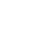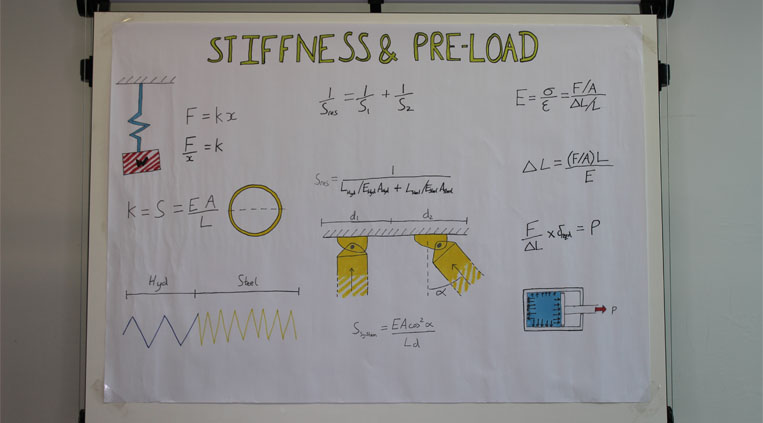# Stiffness & Pre-Load | Back to the Drawing Board

05 Dec# Transcript

Hello and welcome back to the drawing board with me Elliot Griffin. Today I'm going to talk to you about stiffness and preload. Stiffness is a measure of a members resistance to deformation and we're going to focus mainly on axial stiffness but the other ones that you may come across are bending and torsional or stiffness. Pre loading is something that we use to mitigate deflection of our props when they're in the ground. So stiffness in its simplest form can be described like a spring and you may recognize this diagram from an experiment that you might have done in school. It's a simple spring with the weight W on the end and we learn from this that when you apply a weight or a force to a spring that it deflects by x-mount distance. K is described as the spring constant or the spring stiffness of this system. Then we later learn that K can be defined not just by experimentation well it's a product of the cross sectional area of a member multiplied by the young's modulus of the material that that members formed from and divded by the length of the member.  This then gives you the stiffness of a member which can be used to find out how much it will compress or stretch under a given loading.

Now in hydraulic propping it's not simple as a single spring, Steve's talked in previous videos about the hydraulic column of fluid that's at the end of our props that we use to fine tune the length and this is more often than not a lot less stiff than the rest of the steel extensions in the prop. So we have two springs in series and to calculate the overall stiffness of this prop we have to take the reciprocals of both springs. Now when we apply this formula to here and rearrange to make the resultant stiffness the subject, we end up with 1 divided by all these elements. We've got the length of the hydraulic column the Young’s modulus of the hydraulic fluid which we've obtained from test data and the area of the ram bore and we've also got the length, Young's modulus and the area of the steel extensions that were using in that prop. This then gives you a resultant stiffness for a single prop, which can be used to calculate how much it is going to deflect under a load.